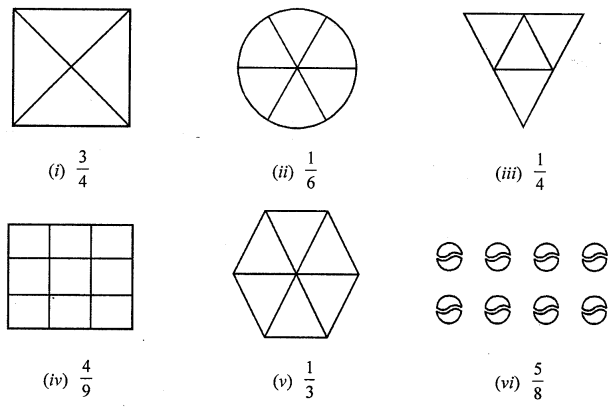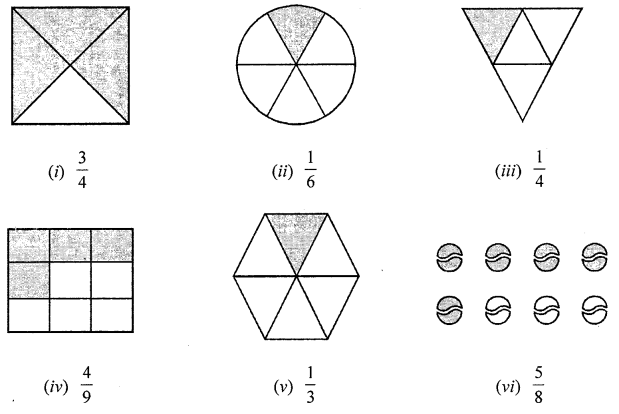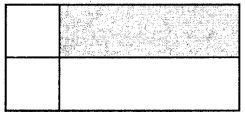## ML Aggarwal Class 6 Solutions for ICSE Maths Chapter 6 Fractions Ex 6.1

Question 1.
Write the following division as fractions:
(i) 3 ÷ 7
(ii) 11 ÷ 78
(iii) 113 ÷ 128
Solution:
(i) 3 ÷ 7 = $$\frac{3}{7}$$
(ii) 11 ÷ 78 = $$\frac{11}{78}$$
(iii) 113 ÷ 128 = $$\frac{113}{128}$$

Question 2.
Write the following fractions in words.
(i) $$\frac{2}{7}$$
(ii) $$\frac{3}{10}$$
(iii) $$\frac{15}{28}$$
Solution:
(i) $$\frac{2}{7}$$ = Two – Seventh
(ii) $$\frac{3}{10}$$ = Three – Tenth
(iii) $$\frac{15}{28}$$ fifteen – Twenty eighth

Question 3.
Write the following fractions in number form:
(i) one – sixth
(ii) three – eleventh,
(iii) seven-fortieth
(iv) thirteen – one hundred twenty fifth
Solution:
(i) One – sixth = $$\frac{1}{6}$$
(ii) Three-eleventh = $$\frac{3}{11}$$
(iiii) seven-forteith = $$\frac{7}{40}$$
(iv) Thirteen-one hundred twenty fifth = $$\frac{13}{125}$$

Question 4.
What fraction of each of the following is shaded part?Solution:Question 5.
Shade the parts of the following figures according to given fractions:Solution:Question 6.
In the adjoining figure, if we say that the shaded region is $$\frac{1}{4}$$ of the whole region, then identify the error in it.Solution:
The whole rectangle is not divided into four equal parts.

Question 7.
Write the fraction in which
(i) numerator = 5 and denominator = 13
(ii) denominator = 23 and numerator = 17
Solution:
(i) $$\frac{5}{13}$$
(ii) $$\frac{17}{23}$$

Question 8.
Shabana has to stitch 35 dresses. So, ar she has stitched 21 dresses. What fraction of dresses has she stitched?
Solution:
Number of dresses she had to stiches = 35
Number of dresses she has finished = 21
∴ Fraction of dresses she has finished = $$\frac{21}{35}=\frac{3}{5}$$

Question 9.
What fraction of a day is 8 hours ?
Solution:
Number of hours in a day = 24 hours
∴ Required fraction = $$\frac{8}{24}$$

Question 10.
What fraction of an hour is 45 minutes ?
Solution:
An hour (1 hour) = 60 minutes
∴ Required fraction = $$\frac{45}{60}$$

Question 11.
How many natural numbers are there from 87 to 97? What fraction of them are prime numbers?
Solution:
The natural numbers from 87 to 97 are 87, 88, 89, 90, 91, 92, 93, 94, 95, 96 and 97. Total number of natural number = 11 Out of these, the prime numbers are 87 and 97
Total number of these prime numbers = 2
∴ Required fraction = $$\frac{2}{11}$$